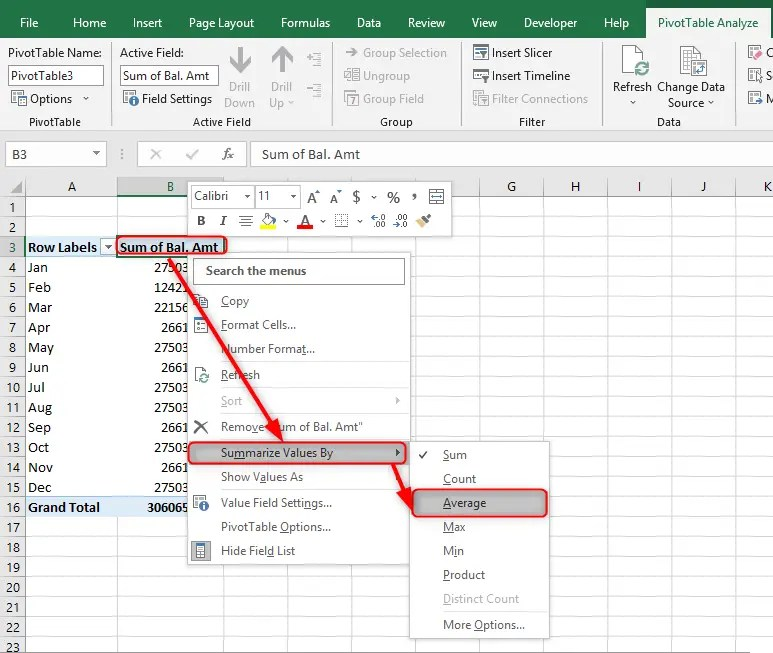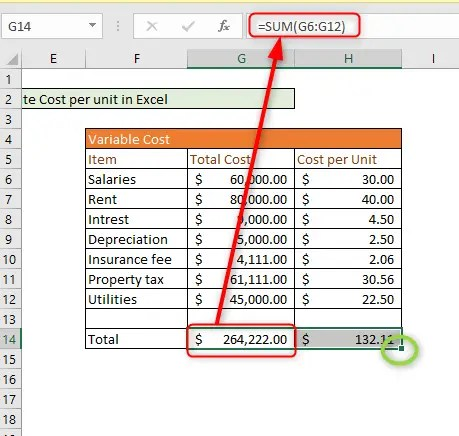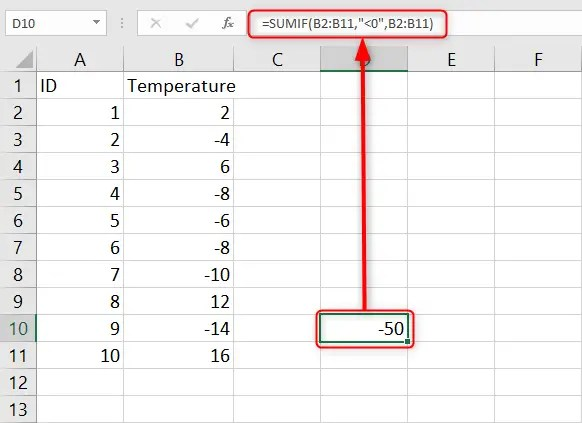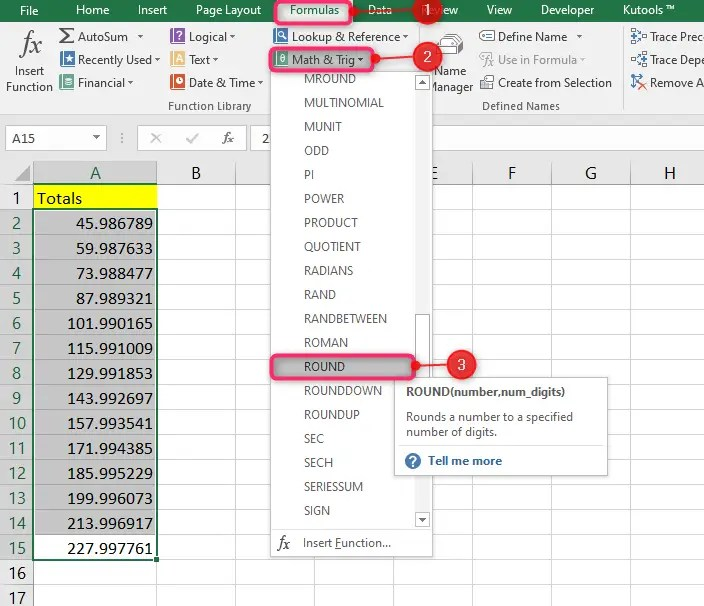# Excel Formulas## How to average per month, week, or day in excel

The article will show you how to do this without going into many details. The best way to calculate these averages is by employing the use of a pivot table. The other ways will involve filtering the table and then calculating the averages one at a time, unlike pivot tables. The pivot tables will just …## How to calculate cost per unit in Excel

In any business, cost per unit calculation is a value calculation. This calculation is defined as a total cost, which includes all costs associated with production, marketing, delivery, warehousing, sales, management, etc., for only a unit of the product. The article will take you through how to do this in excel. Here is the formula …## How to sum negative numbers in Excel

When it comes to Excel’s computation, it is an easier task to perform, as the program provides in-built formulas that make your work easier. Knowing how to apply Excel’s formulas helps you in presenting work that is efficient and reliable. One of the most common computations to do in Excel is additions using the SUM …## How to round time in Excel to the nearest hour (7 Formulas)

Sometimes when working with Microsoft Excel, there may be situations where you may need to round time values. Luckily, Excel offers different rounding functions you can use depending on your purpose. In our guide below, we give step-by-step methods you can use to round time in Excel to the nearest hour. Here we go. Using …## How to calculate in excel (minus)

The use of subtraction is a popular method of calculating using a minus sign. Since the primary level, subtraction has been used regularly, and many are familiar. Apparently, this method also works in Excel. You can use it to subtract a number of things, including percentages, minutes, numbers, hours, seconds, days, and more. Moreover, excel …## How to sum in Excel between sheets

Summing up data is a normal occurrence when dealing with Excel data. Sometimes you may need to sum up data in cells across different or multiple spreadsheets. It is easy enough to do this using Excel’s Sum formula, where you will reference those cells you want to sum.In this guide, we give you steps to …## How to round off in Excel

It is common to come across decimal values when you are working with Excel data. But you can simplify your numerical data by using the ROUND functions. Excel offers its users three ROUND functions, namely: ROUNDUP, ROUND, and ROUNDDOWN. All these functions serve different purposes. Here we discuss how you can round off in Excel …## Excel Formula Vs Excel Function

One of the most confusing terms in Microsoft Excel is Function and Formula. Most people confuse these two features because they perform similar tasks.  But there’s a big difference in how they are designed and how they operate. In this article, I will help you understand the differences between Excel  Formulas Vs Excel Function. What …